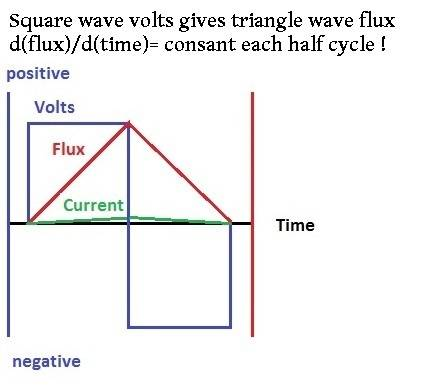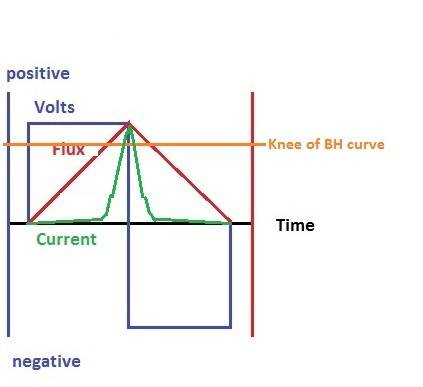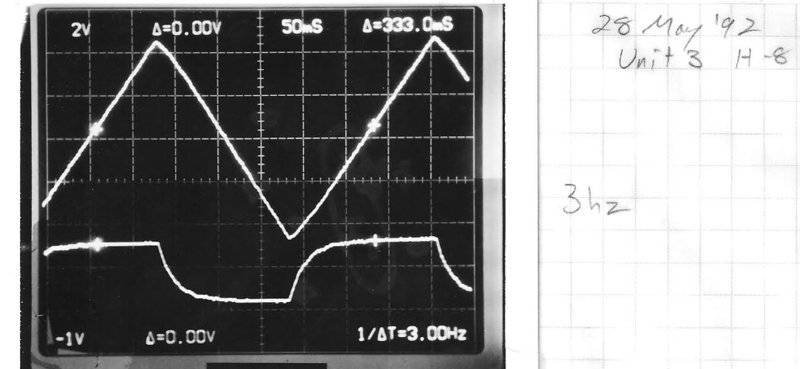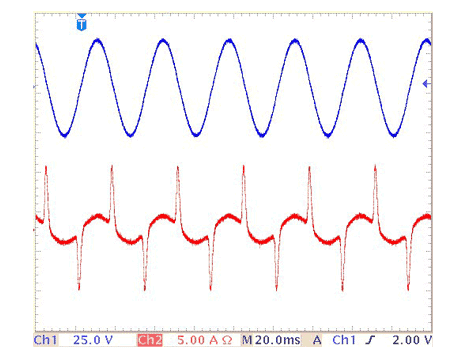# Transformer question: 50Hz versus 60Hz

Will a transformer with 60 Hz being fed to a 50 Hz supply work?

Last edited:

The transformer flux capability is related to the ratio, V/Hz. So for a given voltage rating at 60 Hz, the capability at 50 Hz will be 20% lower, or I mean the voltage at 50 Hz has to be 20% less than the 60 Hz voltage.

So you can't operate with the same voltage rating on 50 Hz as you have at 60 Hz or the core will saturate.

A common 60 Hz voltage is 480 V. That gives you a V/Hz ratio of 8. A common 50 Hz voltage is 400 V, which gives you the same result, so you can use a 480 V 60 Hz transformer on a 50 Hz system if the voltage is 400 V.

a transformer designed for 60 Hz can be supplied by 50 Hz as long as the V/Hz ratio is preserved.

In other words, V60/60 = V50/50

where V60 is the 60 Hz voltage and V50 is the 50 Hz voltage

To preserve the ratio, V50 = 5/6 * V60

so the 50 Hz voltage has to be 83% of the 60 Hz value.

Going the other way, a transformer designed for 50 Hz will have the result

V60 = 6/5 * V50

or - 1.2 * V50

So a 50 Hz transformer can definitely handle the same voltage at 60 Hz.

•jojo13

Ahh I get it. So no matter what, a 50 Hz can be fed to a 60 Hz

Last edited:
Correct!

•jojo13
jim hardy
Gold Member
Dearly Missed
Ahh I get it. So no matter what, a 50 Hz can be fed to a 60 Hz

The why behind it is

A transformer core is sized to carry the magnetic flux necessary to support the voltage you're going to impress on its windings.
Since in any inductor the relation between volts and flux is a derivative-integral one,
volts = -number of turns X d(flux)/d(time) ..... or flux = 1/n X ∫volts X d(time)
volts per turn is a measure of the rate of change of flux in the inductor

It is most easily visualized with a square wave voltageAs the equations show, the integral volt-seconds is a measure of the magnitude of that flux at any instant.
See that flux increases while voltage is positive and decreases while it's negative? That's Mother Nature at work doing real world integrating. Maths Rule !

Okay, so the longer voltage stays positive the higher flux rises.And vice versa.
On a 60 hz power system voltage stays positive for 8.33 milliseconds, a half cycle With a 100 volt square wave that'd be 0.833 volt-seconds.
On a 50 hz power system voltage stays positive for 10 milliseconds. With a hundred volt wave that'd be 1 volt second.
Some power inductor datasheets give the volt-second rating.

Point is the core must be large enough to hold all that flux.
If it's not , excessive magnetizing current will flow trying to force more flux through a core that's already full. That heats both the iron core and the copper windings. You'll hear the core 'buzz' from magnetorestriction.

here's a picture i drew for another thread some years ago..
This core is "full of flux" at the orange line.
Pushing flux all the way up to the required volt-seconds requires excessive magnetizing current and will soon overheat that inductor.I hope that plants the basic idea and sparks an interest in inductance for you.

Train your brain to think in terms of derivative-integral relationships. Mother Nature loves them and it'll help you appreciate the magnificent world she's built around us..

Now in a power system you'll have sine waves not square.
Derivative of sine is cosine which has same shape , so it is not obvious by just looking that integration-differentiation is at play.
That's why i prefer to explain with square and triangle waves it makes it obvious to the eye.
Here's a 'scope trace of a real inductor with triangle wave current forced through it(top trace) - observe voltage is almost square(bottom trace).
That core was rather a poor one hence the rounded corners on the voltage waveHere's an image of a transformer's current when it enters saturation. That could happen from using a 60 hz transformer at 50 hz if you don't decrease applied voltage. (image courtesy of https://www.rane.com/note159.html check it out )Observe current peaks not at voltage peak , but after it when volt-seconds reach the limit of the core.
That transformer will soon be in trouble. I guarantee it's buzzing.

I hope the above long, non-mathematical tome helps someone 'see the light'. That 'scope trace was an "AHA! moment" for my technician and me.
When your intuition leads you to the same formula that's in your textbook it's the start of real understanding.

old jim

#### Attachments

Last edited:
•CalcNerd, Fisherman199, Baluncore and 5 others
anorlunda
Staff Emeritus
Thanks Old Jim. We all love learning from you.

•Fisherman199, davenn and jim hardy
jim hardy
Gold Member
Dearly Missed
Thanks @anorlunda

if i can help somebody relate those erudite derivations in their textbooks to the physical goings on it is worthwhile.

Thank you guys for tolerating this math challenged old fossil.

old jim

anorlunda
Staff Emeritus
••